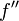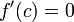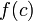# Difference between revisions of "Second derivative test"

## Statement

### What this test is for

This test is a partial test (i.e., it may be inconclusive) for determining whether a given critical point for a function is a point of local minimum, point of local maximum, or neither.

### What the test says

Suppose$f$ is a function and$c$ is a point in the interior of the domain of$f$, i.e.,$f$ is defined on some open interval containing$c$. Suppose, further, that$f''$, i.e., the second derivative of$f$, exists at$c$. Suppose also that$f'(c)=0$, so$c$ is a critical point for$f$. Then:

Hypothesis Conclusion$f''(c) < 0$$f$ attains a local maximum value at$c$ (the value is$f(c)$)$f''(c) > 0$$f$ attains a local minimum value at$c$ (the value is$f(c)$)$f''(c) = 0$ The test is inconclusive.$f$ may attain a local maximum value, a local minimum value, have a point of inflection, or have some different behavior at the point$c$.

### Relation with critical points

The second derivative test is specifically used only to determine whether a critical point where the derivative is zero is a point of local maximum or local minimum. Note in particular that:

• For the other type of critical point, namely that where$f'$ is undefined, the second derivative test cannot be used.
• Since point of local extremum implies critical point, we don't have to worry about points that are not critical points -- none of them will give local extrema.

## Strength of the test

### Second derivative test requires twice differentiability at but not around the point

The second derivative test can be applied at a critical point$c$ for a function$f$ only if$f$ is twice differentiable at$c$. This in particular forces$f$ to be once differentiable around$c$.

However, the test does not require$f''$ to be defined around$c$ or to be continuous at$c$.